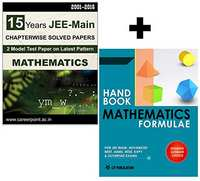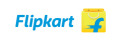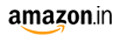# 15 Years JEE Main Mathematics Chapter Wise Solved Papers (2016-2001) + Mathematics Formulae Book JEE MainM.R.P 480 /-400.00 -480.00 -
Product Description

This becomes more important in the context of JEE Main where there is neck-to-neck race. For thIs purpose we feel great pleasure to present before you this book.We have made an attempt to provide 15 Years JEE Main Mathematics chapter wise questions asked in JEE Main from 2001 to 2016 along with solutions.

Features

• Topic-wise collection of past JEE-Main
• Each chapter divides the questions into categories (as per the latest JEE Main pattern) - MCQ single correct answer, MCQ with multiple correct answers, Passage Based, Assertion-Reason, Integer Answer, Fill in the Blanks, True/False and Subjective Questions
• Solutions have been given with enough diagrams, proper reasoning to for better understanding.
• Solutions have been given with enough diagrams, proper reasoning to for better understanding.
• Students must attempt these questions immediately after they complete unit in their class/school/home during preparation

Chapters

1. Functions
2. Limits
3. Continuity & Differentiability
4. Differentiation
5. Tangent & Normal
6. Monotonicity
7. Maxima & Minima
8. Indefinite Integration
9. Definite Integration
10. Area under the curve
11. Theory of Equations
12. Differential Equation
13. Point & Straight Lines
14. Circle
15. Parabola
16. Ellipse
17. Hyperbola
18. Vectors
19. Three Dimensional Geometry
20. Trigonometry
21. Mathematical Reasoning
22. Sequences & Series
23. Measure of Central Tendency
24. Complex Numbers
25. Permutation & Combination
26. Binomial Theorem
27. Probability
28. Determinants
29. Matrices
30. Set & Relation